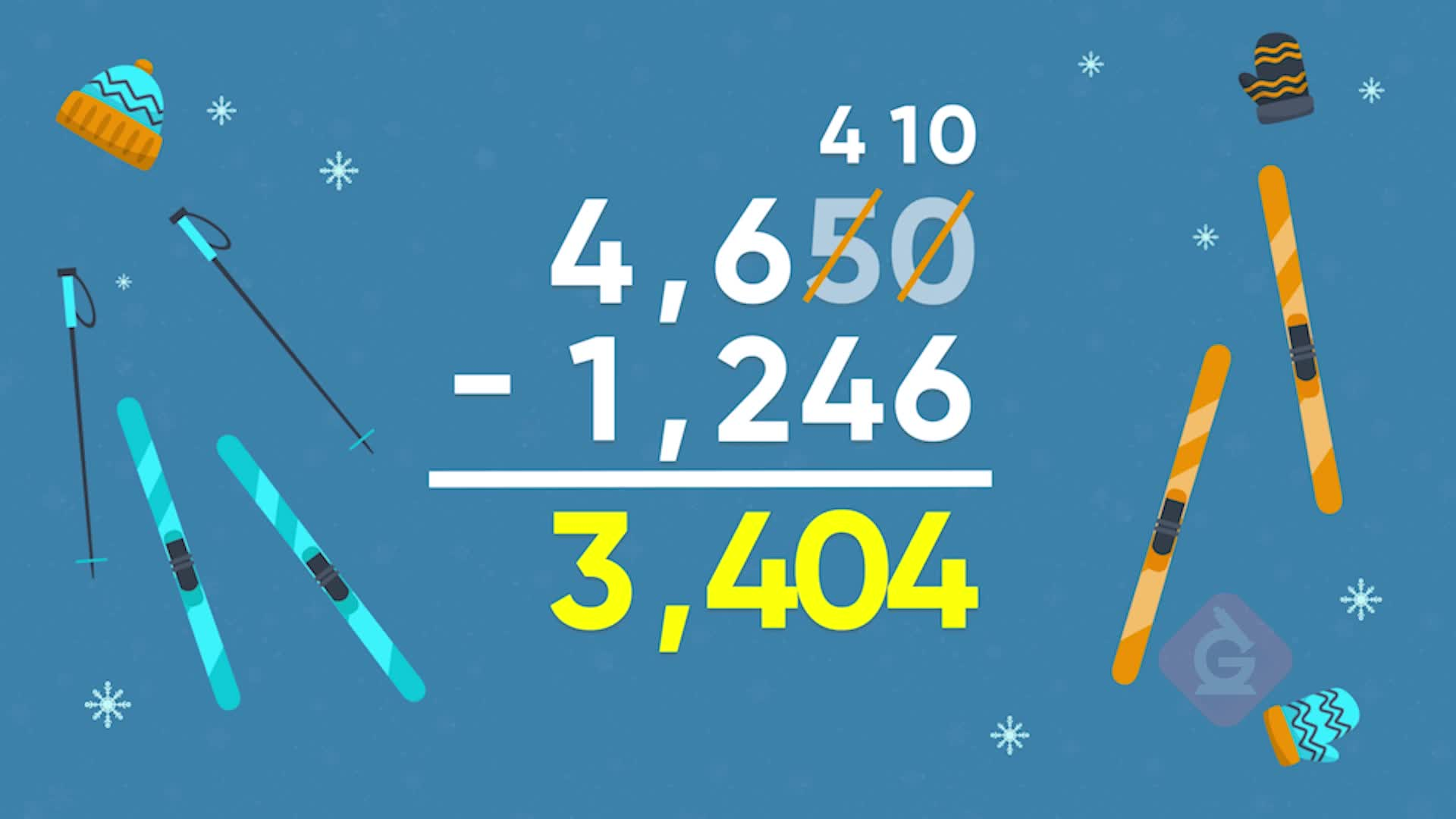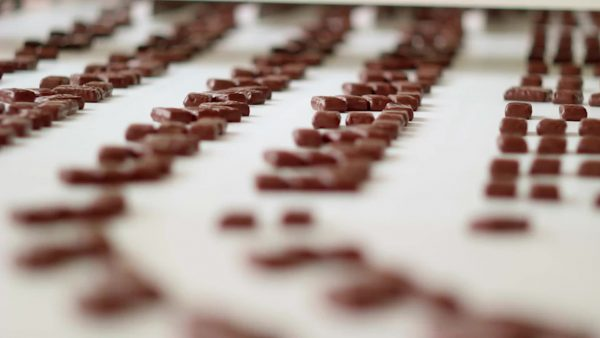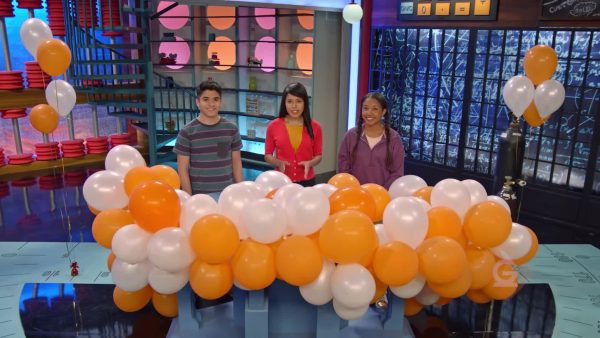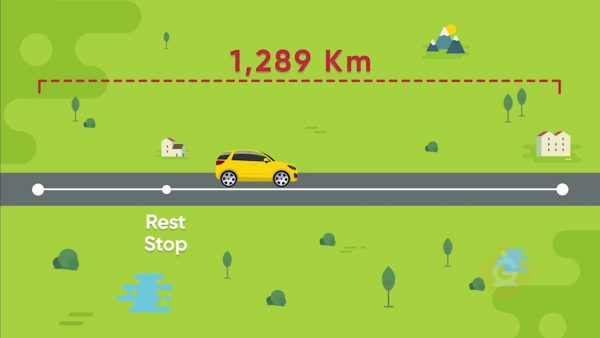Add & Subtract Using the Standard Algorithm Math Videos - Grades 3-5
1%
It was processed successfully!WHAT IS A STANDARD ALGORITHM?

An algorithm is a process or a set of steps to follow to complete a task. The standard algorithms for addition and subtraction are sets of steps you can follow to find any sum or difference of whole numbers.

To better understand adding and subtracting using the standard algorithm…

WHAT IS A STANDARD ALGORITHM?. An algorithm is a process or a set of steps to follow to complete a task. The standard algorithms for addition and subtraction are sets of steps you can follow to find any sum or difference of whole numbers. To better understand adding and subtracting using the standard algorithm…

## LET’S BREAK IT DOWN!Let's say that you bought 4,513 milk chocolate bars and 2,422 dark chocolate bars. You want to know the total number of chocolate bars you bought. To find out, you can write the equation 4,513 + 2,422 = ? and solve it using the standard algorithm. The first step in the standard algorithm is to stack the numbers one on top of the other, making sure they are lined up by place value. Next, we add the ones place: 3 + 2 = 5, and write 5 below the ones column. Next we add the tens column, 1 ten + 2 tens = 3 tens, or 10 + 20 = 30, so we write 3 in the tens column. In the hundreds place, we add 5 + 4 = 9, or 5 hundreds + 4 hundreds = 9 hundreds, and we write 9 in the hundreds column. And finally, we add the thousands place, 4 thousands + 2 thousands = 6 thousands, and write 6 in the thousands column. Now we know that we have 6,935 total chocolate bars. Try this one yourself. One big jar of jellybeans has 3,235 jellybeans and another jar has 1,453 jellybeans. How many jellybeans are there in all?

Adding Candies Let's say that you bought 4,513 milk chocolate bars and 2,422 dark chocolate bars. You want to know the total number of chocolate bars you bought. To find out, you can write the equation 4,513 + 2,422 = ? and solve it using the standard algorithm. The first step in the standard algorithm is to stack the numbers one on top of the other, making sure they are lined up by place value. Next, we add the ones place: 3 + 2 = 5, and write 5 below the ones column. Next we add the tens column, 1 ten + 2 tens = 3 tens, or 10 + 20 = 30, so we write 3 in the tens column. In the hundreds place, we add 5 + 4 = 9, or 5 hundreds + 4 hundreds = 9 hundreds, and we write 9 in the hundreds column. And finally, we add the thousands place, 4 thousands + 2 thousands = 6 thousands, and write 6 in the thousands column. Now we know that we have 6,935 total chocolate bars. Try this one yourself. One big jar of jellybeans has 3,235 jellybeans and another jar has 1,453 jellybeans. How many jellybeans are there in all?

### Decorating with BalloonsLet's say you are decorating the school gym. You put up 3,578 balloons in the morning and another 2,212 balloons in the afternoon. You want to know how many balloons you put up in all. You can find out by adding 3,578 + 2,212 using the standard algorithm. Stack the number 3,578 on top of 2,212, making sure the digits are lined up by their place value and begin adding with the ones column. First we add 8 ones + 2 one = 10 ones. Since we have a sum of 10 ones, these can be regrouped as 1 tens. Write 0 below the ones column and 1 above the 7 in the tens place in the top number. We can't forget to add this 1 ten that we regrouped when we add the tens column! Next, we add the tens column to get 1 ten + 7 tens + 1 ten = 9 tens, and we write 9 in the tens column. Next, we add 5 hundreds + 2 hundreds = 7 hundreds, and write a 7 below the hundreds column. Finally, we add 3 thousands + 2 thousands = 5 thousands, and write a 5 in the thousands place. We've now answered our question: you put up 5,790 balloons in all. Try this one yourself. You ride your bike 3,242 meters to the store and another 739 meters to your friend's house. How many total meters did you ride?

Decorating with Balloons Let's say you are decorating the school gym. You put up 3,578 balloons in the morning and another 2,212 balloons in the afternoon. You want to know how many balloons you put up in all. You can find out by adding 3,578 + 2,212 using the standard algorithm. Stack the number 3,578 on top of 2,212, making sure the digits are lined up by their place value and begin adding with the ones column. First we add 8 ones + 2 one = 10 ones. Since we have a sum of 10 ones, these can be regrouped as 1 tens. Write 0 below the ones column and 1 above the 7 in the tens place in the top number. We can't forget to add this 1 ten that we regrouped when we add the tens column! Next, we add the tens column to get 1 ten + 7 tens + 1 ten = 9 tens, and we write 9 in the tens column. Next, we add 5 hundreds + 2 hundreds = 7 hundreds, and write a 7 below the hundreds column. Finally, we add 3 thousands + 2 thousands = 5 thousands, and write a 5 in the thousands place. We've now answered our question: you put up 5,790 balloons in all. Try this one yourself. You ride your bike 3,242 meters to the store and another 739 meters to your friend's house. How many total meters did you ride?Let's say you are taking a road trip to visit a friend who lives 1,289 km from where you live. After traveling 112 km you stop at a rest stop. Now you would like to know how much farther you have to travel to your destination. You can answer this by using the standard algorithm to solve 1,289 km – 112 km = how many km? We stack the numbers one on top of the other with the first number on top and the digits aligned by place value. We then subtract, beginning with the ones column. 9 – 2 = 7, so write 7 below the ones column. In the tens place we subtract 8 tens – 1 ten = 7 tens, and we write a 7 below the tens column. In the hundreds place we have 2 hundreds – 1 hundred = 1 hundred, and write 1 below the hundreds column. Finally, in the thousands place, we have 1 thousand – nothing, which is the same as 1 thousand – 0 thousands = 1 thousand, so we write 1 below the thousands column. You have another 1,177 km to travel. Try this one yourself. Researchers have collected 4,523 samples of soil near the ocean and 1,721 samples of soil near the lake. How many more samples of soil near the ocean than samples of soil near the lake have they collected?

Road Trip Let's say you are taking a road trip to visit a friend who lives 1,289 km from where you live. After traveling 112 km you stop at a rest stop. Now you would like to know how much farther you have to travel to your destination. You can answer this by using the standard algorithm to solve 1,289 km – 112 km = how many km? We stack the numbers one on top of the other with the first number on top and the digits aligned by place value. We then subtract, beginning with the ones column. 9 – 2 = 7, so write 7 below the ones column. In the tens place we subtract 8 tens – 1 ten = 7 tens, and we write a 7 below the tens column. In the hundreds place we have 2 hundreds – 1 hundred = 1 hundred, and write 1 below the hundreds column. Finally, in the thousands place, we have 1 thousand – nothing, which is the same as 1 thousand – 0 thousands = 1 thousand, so we write 1 below the thousands column. You have another 1,177 km to travel. Try this one yourself. Researchers have collected 4,523 samples of soil near the ocean and 1,721 samples of soil near the lake. How many more samples of soil near the ocean than samples of soil near the lake have they collected?

### Cross-Country SkiingLet's say you are cross-country skiing on a trail that is 4,650 meters long and you have skied 1,246 meters so far. How much farther do you have to ski before reaching the end of the trail? To answer this we can solve the equation 4,650 – 1,246 = ? using the standard algorithm for subtraction. Stack 4,650 on top of 1,246, making sure the place values are lined up. Beginning at the ones place, we have 0 ones – 6 ones, which we cannot do since 0 is smaller than 6. We need to regroup 1 ten from the tens place. When we regroup from the tens place to the ones place, we make 5 tens in the top number 4 tens, and then 0 ones is now 10 ones. We can now subtract 10 ones – 6 ones = 4 ones, and write 4. We now subtract the tens column, 4 tens – 4 tens = 0 tens, and write 0 in the tens column. In the hundreds column, we subtract 6 hundreds – 2 hundreds = 4 hundreds. And in the thousands place, we have 4 thousands – 1 thousand = 3 thousands. We have another 3,404 meters to ski to get to the end of the trail. Try this one yourself. You eat 2,200 calories a day and your younger sister eats 1,235 calories a day. How many more calories do you eat than your sister each day?

Cross-Country Skiing Let's say you are cross-country skiing on a trail that is 4,650 meters long and you have skied 1,246 meters so far. How much farther do you have to ski before reaching the end of the trail? To answer this we can solve the equation 4,650 – 1,246 = ? using the standard algorithm for subtraction. Stack 4,650 on top of 1,246, making sure the place values are lined up. Beginning at the ones place, we have 0 ones – 6 ones, which we cannot do since 0 is smaller than 6. We need to regroup 1 ten from the tens place. When we regroup from the tens place to the ones place, we make 5 tens in the top number 4 tens, and then 0 ones is now 10 ones. We can now subtract 10 ones – 6 ones = 4 ones, and write 4. We now subtract the tens column, 4 tens – 4 tens = 0 tens, and write 0 in the tens column. In the hundreds column, we subtract 6 hundreds – 2 hundreds = 4 hundreds. And in the thousands place, we have 4 thousands – 1 thousand = 3 thousands. We have another 3,404 meters to ski to get to the end of the trail. Try this one yourself. You eat 2,200 calories a day and your younger sister eats 1,235 calories a day. How many more calories do you eat than your sister each day?

## ADD & SUBTRACT USING THE STANDARD ALGORITHM VOCABULARY

Finding the sum of two or more numbers.
Sum
Subtraction
Find the difference between two numbers.
Difference
The result of subtraction.
Place value
A system that gives a digit a value according to its position, or place, in a number. In our base-ten system for writing numbers, each place has a value 10 times that of the place to its right.
Expanded form
A way of writing a number as the sum of the values of each digit.
Exchanging 10 in one place-value position for 1 in the position to the left in order to carry out addition, or exchanging 1 for 10 in one place-value position to the right for the purpose of carrying out subtraction.
A step-by-step method for addition and subtraction of multi-digit numbers based on place value and regrouping.

## ADD & SUBTRACT USING THE STANDARD ALGORITHM DISCUSSION QUESTIONS

### What are the steps to the standard algorithm for addition?

1) Stack the numbers vertically by place value.
2) Add together the numbers that share the same place value, beginning in the ones column.
3) Write the sum below each column.
4) If the sum in a column is greater than 9, regroup.

### What are the steps to the standard algorithm for subtraction?

1) Stack the numbers vertically by place value with the first number on top.
2) Subtract the numbers that share the same place value, beginning in the ones column.
3) Write the difference below each column.
4) If the top number in a column is less than the bottom number, regroup before subtracting.

### Marcos says that 3,743 + 128 = 3,861. Is he correct? Why or why not?

He is incorrect. He added 3 + 8 = 11 in the ones place and put a 1 in the ones column but forgot to regroup 10 ones to 1 ten in the tens place.

### Hailey finds that 4,005 – 2,238 = 2,233. Is she correct? Why or why not?

She is incorrect. She subtracted the smaller number from the bigger number in each column, not the bottom number from the top.

### Can the standard algorithm be used for all numbers of a given size or be extended to larger numbers?

Yes, the standard algorithm can be used for any addition or subtraction problem.
X

## Success

We’ve sent you an email with instructions how to reset your password.
Ok

## Choose Your Free Trial Period### 7 DaysContinue to Lessons### 90 DaysGet 90 days free by inviting other teachers to try it too.

Share with Teachers

## Get 90 Days Free

### By inviting 4 other teachers to try it too.

4 required

Skip, I will use a 7 days free trial

## Thank You!

Enjoy your free 90 days trial